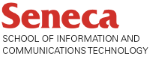# DPS923 & MAP523

Mobile App Development for iOS

## How to: Substring

In other languages, there is typically “substring” functionality in a string class.

Swift does this differently.

### Background info

A string object obviously uses space in memory.

Interestingly, a Swift substring does NOT use space in memory. Instead, it points to a range of characters in the original string.

### Substrings

Let’s look at some common scenarios.

#### First “x” characters

Assume that we want the first five characters of a string. Here’s how.

``````let str = "Hello, world!"
// str is a String instance

let part1 = str.prefix(5)
// part1 is a String.SubSequence instance,
// and points to the first 5 characters of str
``````

Before using `part1` as a string, we must convert it:

``````let part1string = String(part1)
// part1string is a new String instance
``````

The diagram below shows this procedure:

• A string was created, containing “Hello, world!”
• A substring was created, pointing to the first five characters
• A new string was created by converting the substring to a string#### All except first “x” characters

Assume that you want all except the first seven characters:

``````let str = "Hello, world!"
// str is a String instance

let part2 = str.dropFirst(7)
// part2 is a String.SubSequence instance,
// and points to the str after the first 7 characters

let part2string = String(part2)
// part2string is a new String instance
``````

#### Last “x” characters

Assume that you want the last six characters:

``````let str = "Hello, world!"
// str is a String instance

let part3 = str.suffix(6)
// part3 is a String.SubSequence instance,
// and points to the last 6 characters of str

let part3string = String(part3)
// part3string is a new String instance
``````

#### Character range in the midst of a string

We must use indexes (start and end) to help with this task.

Assume the following string:

``````let fox = "The quick brown fox jumped over the lazy dog."
``````

Then, assume that we want only the text “jumped over”. There are several how-to strategies (including using some of the techniques above), but assume that we are NOT going to count characters. Instead, were going to use sub-string searching.

Here’s one way to get it:

``````// Find the starting index of "jumped over"
// Here, assume it WILL find what it's looking for
let range = fox.range(of: "jumped over")!
// range is an instance of Range, a start-to-end structure
let part4string = fox[range]
// part4string is a new String instance
``````

#### Does “string” contain “substring”?

Use the `contains()` method, which returns a boolean:

``````let fox = "The quick brown fox jumped over the lazy dog."
let hasJump = fox.contains("jumped over")
// hasJump will be true
``````

#### Replace part of a string

Use the `replacingOccurrences()` method on the original string, which returns a new string:

``````let fox = "The quick brown fox jumped over the lazy dog."
let goat = fox.replacingOccurrences(of: "brown fox", with: "grey goat")
// goat is a new String instance
``````

The Swift Substring structure has much functionality. Look at the reference documentation for more.

And remember to look at the Swift Documentation section on strings.